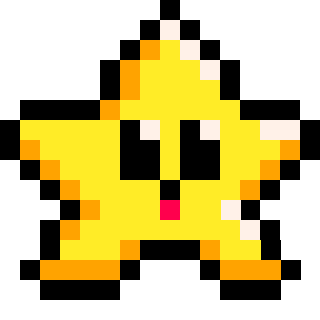# NUMBER

A number is a type of variable that holds a numerical value.

That's a very specific way of saying a number is a number, but it is important to understand that in code, a number can be stored in different ways, and that there is a difference betweeen `5` and `"5"`. Numbers can be integers, floats, negative, or positive:

``````a = 10      --number value as integer
b = 10.567  --number value as float
c = -10     --number value as negative
d = "10"    --string value, not a number``````

Even though the string `d` in the example holds the digits "1" and "0", it is not actually a number when we are talking about variable types.

#### Converting between Numbers and Strings

PICO-8 is able to assume when it should automatically convert strings to numbers or numbers to strings:

``````string = "10"
num = 5

print( string + num )   --converted twice
``````

In the above example, we are trying to add a string with a number. Usually, this would give an error: `Attempt to perform arithmetic on a string value.` But if it can, PICO-8 will convert the string to a number first so that it can properly add the two number type variables `10+5`. But it's not finished yet. The `print()` function requires strings not numbers, so PICO-8 will also convert the sum back into a string so that it can be printed to the screen.

However, this doesn't always work as you might expect, so be careful not to rely on this automatic conversion too much.

For example, it is common to want to print a line of text that has both numbers and strings. To do this, you must use the `..`operator to concatenate the different types of variables into a string. For example:

``````string = "score= "
num = 50

print( string num )  --error

print( string..num ) --prints: score= 50
``````257

26 May 2023

`Font`